## Class definitions

Before we can use classes, we have to define them. Assume the following simple class:

Figure 11.1. Hello World ClassIn C++ there are two parts to every class:

• Class definition

• Class implementation

### Important

Class definition

The class definition defines the structure of the class. It defines the class name, member variables and member functions.

Class implementation

The class implementation specifies the behavior of the class. It gives an implentation for its member functions.

Warning to all tha have used Java before: In Java they are both together, in C++ they have to be separated!

Sidenote: As you should be able to guess, pure virtual classes (interfaces) have no implementation.

But back to the class. The first step is redefining it for the actual implementation (we learned some of that earlier).

• Use getters / setters instead of public attributes

• Use Vector<> for multiplicity

In an actual project we would probably do this step in our head. But it doesn't hurt do to on paper:

Figure 11.2. Hello redefined for implementationAlthough it is technically possible and perfectly legal to declare public attributes in C++, it is not legal in this class! For all projects, designs, implementations, etc. you do in this class you have to use private attributes!

The example here is intentionally simple. In reality we would probably declare a default value for formal, but we need to know about constructors first (in one of the next classes).

Given the definition in UML we can now translate it into C++.

Example for a class definition:

```class Hello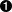{
private: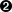bool formal;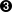public: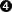void greeting();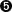bool getFormal();
void setFormal(bool f);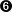};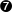```

Lets look at the different parts:

 The class definition starts with the keyword "class" followed by the class name, followed by an open brace (similar to structs). Visibility modifiers in C++ are a given as the keyword (private or public) and then a colon (:). Note that there is no "boolean" datatype in C++, it is called "bool". Other than that an attribute defintion is similar to a variable definition. Visibility modifiers count for more than just the next definition. They count for every definition from that point onward until the end of the class (unlike Java). A function with no return has the "void" return type. Member function definitions look like C++ prototype declarations. Remember, in C++ it is type, space, name The class definition ends with a closed brace and a semicolon! Do not forget the semicolon! (This is the same as with structs).

Other notes

Order

As in UML, in C++ it is convention to declare all variables first, then all member functions.

Indentation

Usually the class definition starts with no indentatation. Visibility modifiers and the closing brace are on the same level as the class definition. Member attributes and operations are indented.

As you can see a C++ class definition shows the exact same thing as a UML class definition. This is not a coincidence. There are even programs that can produce one from the other. However, these are very expensive.

Practice:

Write a C++ class definition for this class:

Figure 11.3. Polygon classHints: You will not need any getters / setters here. The corrent type for "points" would be "Vector<Location>".

We have now defined a class and its interface. But now we have to give actual implementations for the defined methods.

Lets look at the Hello class again:

Figure 11.4. Specification for helloWe have defined the class

And its attributes.

What is missing is the methods.

Fortunately, implementing the methods is much like we've seen in implementations before:

```void Hello::greeting()
{
if (formal)
cout << "Hello, nice to meet you!" << endl;
else
cout << "What's up?" << endl;
}

...```

The "Hello::" is borrowed from namespaces. In this case, a class is somewhat like a namespace (although a different thing)

The header line of a method implementation is:

Return data type (void if no return value), class name, colon-colon (::), method name, parameters

The body of a method is exactly the same as we learned in earlier.

In the method, we can make use of all attributes of the same class as if they were global variables. In the example given we can use "formal" because it is defined inside the class "Hello" and our greeting method is for the class "Hello".

Practice: Assume the following class definition:

```class Point
{
private:
float x;
float y;
public:
float distanceFromOrigin();
};```

Give an implementation for the distanceFromOrigin function. Note: the formula is root(x^2+y^2) (our course this is NOT C++ notation).